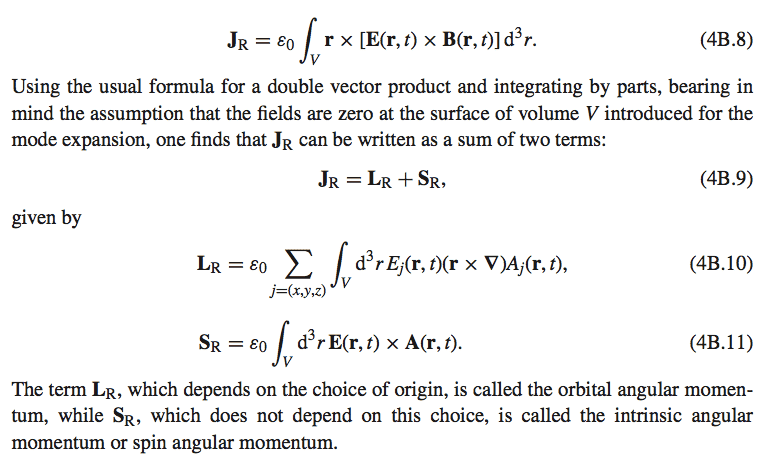# Vector integration by parts

• II was trying to derive the following results from 4B.8 as suggested by using the vector triple product identity but have been unsuccessful in deriving ##\vec{L_R}## and ##\vec{S_R}## in the end. After using the identity and finding the integrand to be ## \vec{E}(\vec{r}\cdot\vec{B}) - \vec{B} (\vec{r} \cdot \vec{E}) ## what would be the next best step to take to re-derive the 4B.10 and 4B.11?

After using the identity and finding the integrand to be ## \vec{E}(\vec{r}\cdot\vec{B}) - \vec{B} (\vec{r} \cdot \vec{E}) ##
I haven't tried it, but my guess is that this is not the right identity to use, and that you should rather use the Jacobi identity that is a couple of lines below in the Wikipedia page.

I haven't tried it, but my guess is that this is not the right identity to use, and that you should rather use the Jacobi identity that is a couple of lines below in the Wikipedia page.

Okay. I've looked into that identity as well now, and after using it, still don't quite get any further since it reduces back to the case of the integrand equalling ## \vec{E}(\vec{r}\cdot\vec{B}) - \vec{B} (\vec{r} \cdot \vec{E}) ##.